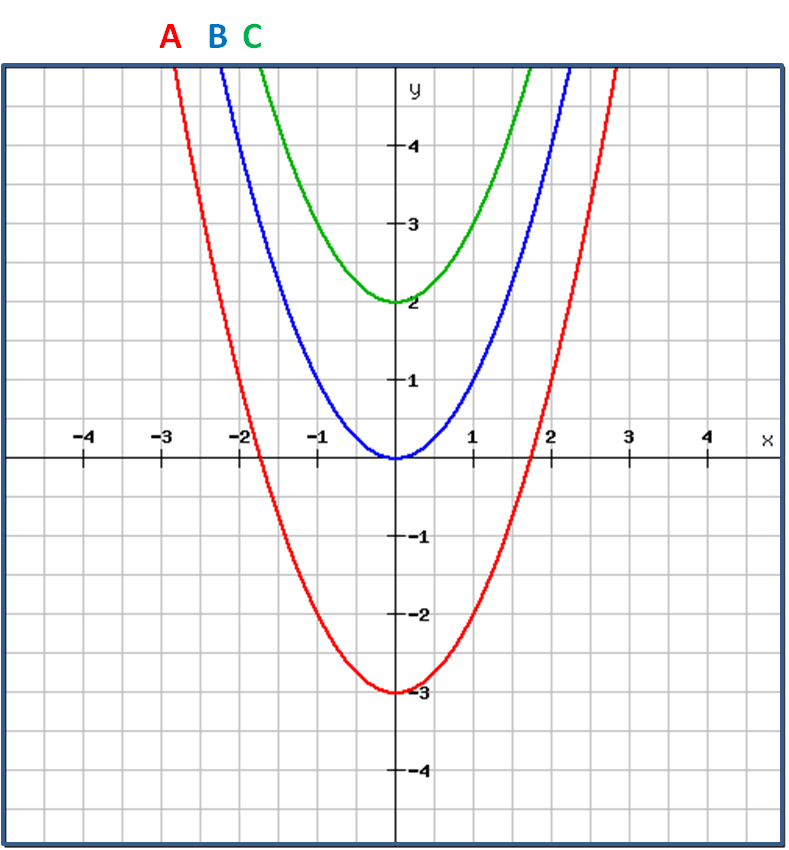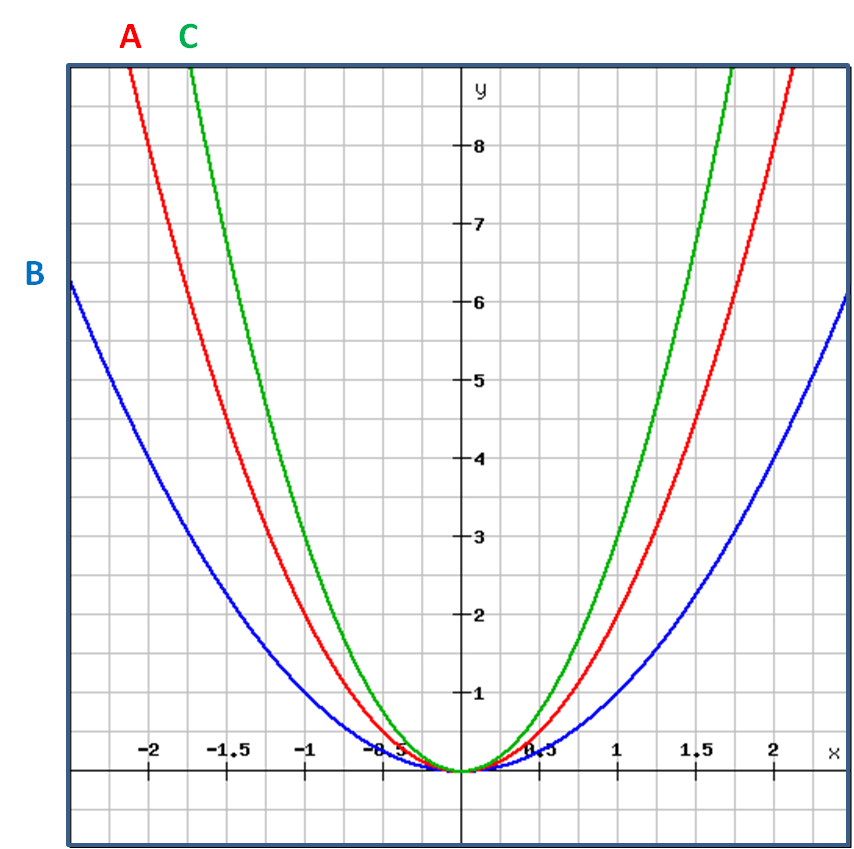# Shifting Curves

In this worksheet, students must identify the equation of a curve after it has been transformed or shifted.Key stage:  KS 4

Curriculum topic:  Algebra

Difficulty level:### QUESTION 1 of 10

This worksheet is about the shapes of quadratic graphs.

All are parabolic but their equations can be used to identify their shapes quickly and easily.

We start with the basic function f(x) = x², or the graph of the parabola y = x².

Vertical shifts

We get equations of the form  y = x² ± kHorizontal shifts

We get equations of the form  y = (x ± k)²Stretching

We get equations of the form  y = kx²What shape are these curves?quadratic

parabolas

hyperbolas

squared

Select the correct equation which is represented by graph B.y = x²

y = 2x²

y = 3x²

y = (x - 3)²

y = (x - 2½)²

y = (x + 1)²

y = x² - 3

y = (x + 3)²

y = x² + 2

Select the correct equation which is represented by graph C.y = x²

y = 2x²

y = 3x²

y = (x - 3)²

y = (x - 2½)²

y = (x + 1)²

y = x² - 3

y = (x + 3)²

y = x² + 2

Select the correct equation which is represented by graph B.y = x²

y = 2x²

y = 3x²

y = (x - 3)²

y = (x - 2½)²

y = (x + 1)²

y = x² - 3

y = (x + 3)²

y = x² + 2

Select the correct equation which is represented by graph A.y = x²

y = 2x²

y = 3x²

y = (x - 3)²

y = (x - 2½)²

y = (x + 1)²

y = x² - 3

y = (x + 3)²

y = x² + 2

Select the correct equation which is represented by graph A.y = x²

y = 2x²

y = 3x²

y = (x - 3)²

y = (x - 2½)²

y = (x + 1)²

y = x² - 3

y = (x + 3)²

y = x² + 2

Select the correct equation which is represented by graph A.y = x²

y = 2x²

y = 3x²

y = (x - 3)²

y = (x - 2½)²

y = (x + 1)²

y = x² - 3

y = (x + 3)²

y = x² + 2

Select the correct equation which is represented by graph B.y = x²

y = 2x²

y = 3x²

y = (x - 3)²

y = (x - 2½)²

y = (x + 1)²

y = x² - 3

y = (x + 3)²

y = x² + 2

Select the correct equation which is represented by graph C.y = x²

y = 2x²

y = 3x²

y = (x - 3)²

y = (x - 2½)²

y = (x + 1)²

y = x² - 3

y = (x + 3)²

y = x² + 2

Select the correct equation which is represented by graph C.y = x²

y = 2x²

y = 3x²

y = (x - 3)²

y = (x - 2½)²

y = (x + 1)²

y = x² - 3

y = (x + 3)²

y = x² + 2

• Question 1

What shape are these curves?CORRECT ANSWER
parabolas
EDDIE SAYS
They are all parabolic. Their equations will have an x² term.
• Question 2

Select the correct equation which is represented by graph B.CORRECT ANSWER
y = x²
EDDIE SAYS
This is the basic y = x² curve.
• Question 3

Select the correct equation which is represented by graph C.CORRECT ANSWER
y = (x + 1)²
EDDIE SAYS
This is the basic y = x² curve shifted 1 unit to the left.
When x = -1, y = 0.
• Question 4

Select the correct equation which is represented by graph B.CORRECT ANSWER
y = x²
EDDIE SAYS
This is the basic y = x² curve.
• Question 5

Select the correct equation which is represented by graph A.CORRECT ANSWER
y = x² - 3
EDDIE SAYS
This is the basic y = x² curve shifted down 3 units.
When x = 0, y = -3.
• Question 6

Select the correct equation which is represented by graph A.CORRECT ANSWER
y = (x - 2½)²
EDDIE SAYS
This is the basic y = x² curve shifted 2½ units to the right.
When x = 2½, y = 0.
• Question 7

Select the correct equation which is represented by graph A.CORRECT ANSWER
y = 2x²
EDDIE SAYS
This is the basic y = x² curve where the x² term has been multiplied by 2.
• Question 8

Select the correct equation which is represented by graph B.CORRECT ANSWER
y = (x + 3)²
EDDIE SAYS
This is the basic y = x² curve shifted 3 units to the left.
When x = -3, y = 0.
• Question 9

Select the correct equation which is represented by graph C.CORRECT ANSWER
y = 3x²
EDDIE SAYS
This is the basic y = x² curve where the x² term has been multiplied by 3.
• Question 10

Select the correct equation which is represented by graph C.CORRECT ANSWER
y = x² + 2
EDDIE SAYS
This is the basic y = x² curve shifted 2 units up.
When x = 0, y = 2.
---- OR ----

Sign up for a £1 trial so you can track and measure your child's progress on this activity.

### What is EdPlace?

We're your National Curriculum aligned online education content provider helping each child succeed in English, maths and science from year 1 to GCSE. With an EdPlace account you’ll be able to track and measure progress, helping each child achieve their best. We build confidence and attainment by personalising each child’s learning at a level that suits them.

Start your £1 trial